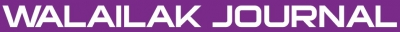A New Analytical Technique to Solve Some Equations Involving Trigonometric Nonlinearities

Abstract

An analytical approximate method for non-linear problems in heat transfer, namely the Homotopy Analysis Method, is employed to propose a new approach for the problem arising in heat transfer with a trigonometric nonlinearity. Numerical example is presented to illustrate the validity and efficiency of the proposed approach. The results reveal that the method is very effective, straightforward and simple.

Keywords

Heat transfer; Homotopy Analysis Method, Symbolic computation, Approximate series solution

PDF

References

E Doedel. Finite difference methods for nonlinear two-point boundary-value problems. SIAM J. Numer. Anal. 1979; 16, 173-85.

AG Deacon and S Osher. Finite-element method for a boundary-value problem of mixed type. SIAM J. Numer. Anal. 1979; 16, 756-78.

G Adomian. Solving Frontier Problems of Physics: the Decomposition Method. Kluwer Academic, Dordrecht, 1994.

JH He. The homotopy perturbation method for nonlinear oscillators with discontinuities. Appl. Math. Comput. 2004; 151, 287-92.

DA Nield and A Bejan. Convection in Porous Media. 2nd ed. Springer, New York, 1998.

SJ Liao. An approximate solution technique not depending on small parameters: a special example. Int. J. Non-Linear Mech. 1995; 30, 371-80.

S Abbasbandy. Homotopy analysis method for heat radiation equations. Int. Comm. Heat Mass Tran. 2007; 34, 380-7.

S Abbasbandy. The application of homotopy analysis method to nonlinear equations arising in heat transfer. Phys. Lett. A 2006; 360, 109-13.

A Rajabi, DD Ganji and H Taherian. Application of homotopy perturbation method in nonlinear heat conduction and convection equations. Phys. Lett. A 2007; 360, 570-3.

SJ Liao. Beyond Perturbation: Introduction to the Homotopy Analysis Method. Chapman & Hall, Boca Raton, 2003.

SJ Liao and Y Tan. A general approach to obtain series solutions of nonlinear differential equations. Stud. Appl. Math. 2007; 119, 297-354.

AD Polyanin and VF Zaitsev. Handbook of Nonlinear Partial Differential Equations. Chapman & Hall/CRC Press, Boca Raton, USA, 2004.

Refbacks

• There are currently no refbacks.Online ISSN: 2228-835X

http://wjst.wu.ac.th

Last updated: 17 May 2019